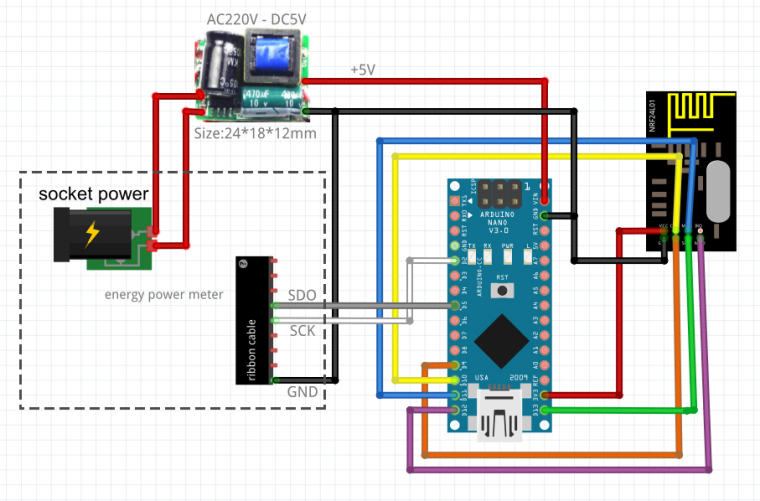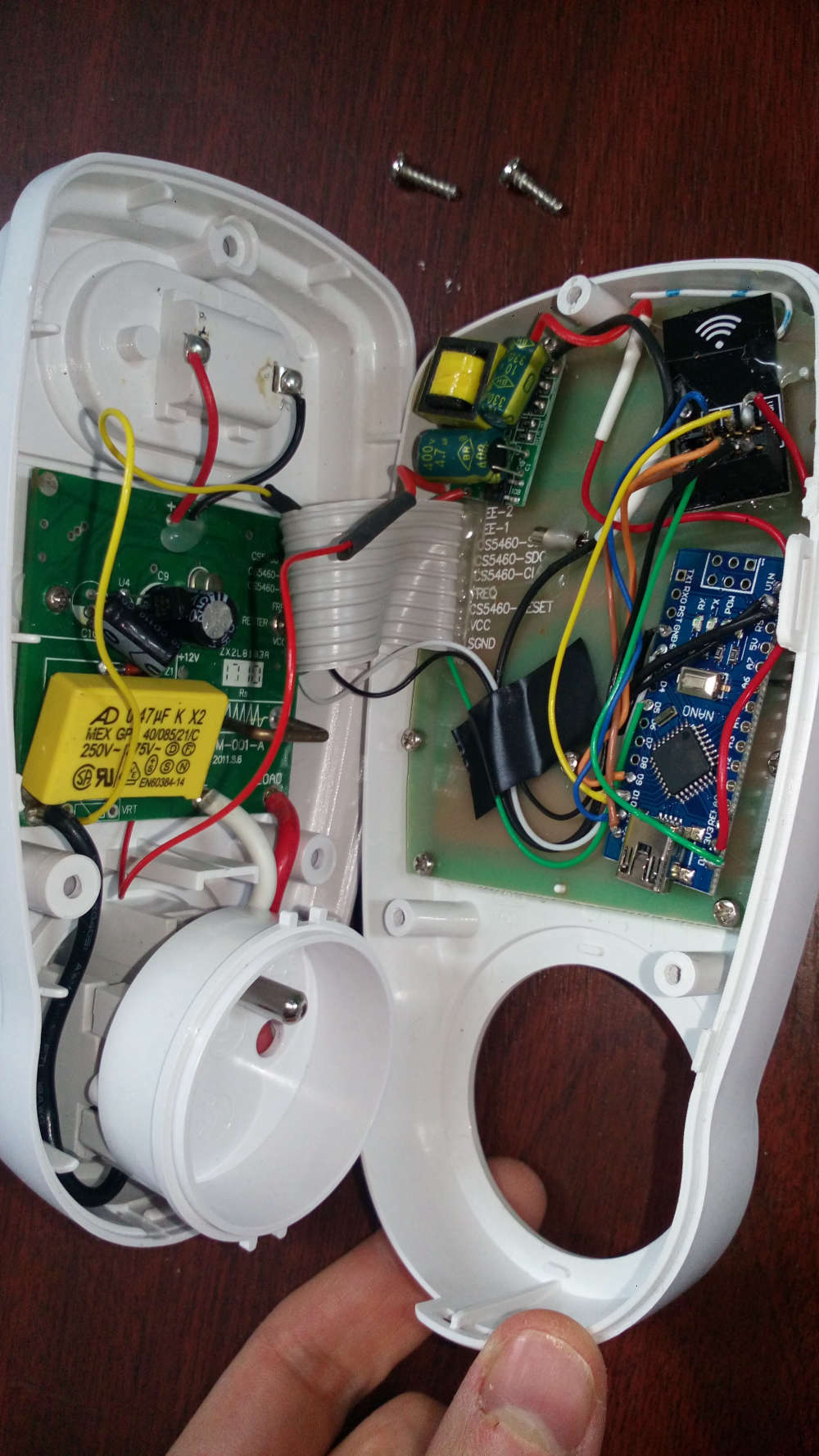Power Meter with product energy power meter socket (based on IC CS5460A)

• Hello,

I would like to use Karl's work on the hack of an energy power meter socket:
http://gizmosnack.blogspot.fr/2014/10/power-plug-energy-meter-hack.html
and add a mysensors node to retrieve the values in Domoticz.
I bought this one: https://www.amazon.fr/GreenBlue-GB202-consommation-dénergie-wattmètre/dp/B00E5BONDU/ref=sr_1_2?ie=UTF8&qid=1510248821&sr=8-2&keywords=wattmetre
It's the same model based on IC CS5460A (datasheet).

I uploaded the sketch of Karl in a Arduino Nano, and i can read the values correctly by displaying them on 16x2 LCD screen.

Good news !
I modified my sketch, and it's OK: the values are read correctly.

My sketch:

//Enable debug prints
//#define MY_DEBUG

// Enable and select radio type attached

#include <MySensors.h>

#include <Wire.h>
#include <LiquidCrystal_I2C.h>

#define BACKLIGHT_PIN 3
#define En_pin 2
#define Rw_pin 1
#define Rs_pin 0
#define D4_pin 4
#define D5_pin 5
#define D6_pin 6
#define D7_pin 7

LiquidCrystal_I2C lcd(0x27,En_pin,Rw_pin,Rs_pin,D4_pin,D5_pin,D6_pin,D7_pin);

//#define CHILD_ID 5              // Id of the sensor child

const int CLKPin = 2; // Pin connected to CLK (D2 & INT0)
const int MISOPin = 5;  // Pin connected to MISO (D5)

//All variables that is changed in the interrupt function must be volatile to make sure changes are saved.
volatile int Ba = 0;   //Store MISO-byte 1
volatile int Bb = 0;   //Store MISO-byte 2
volatile int Bc = 0;   //Store MISO-byte 2
float U = 0;    //voltage
float P = 0;    //power
float ReadData = {0, 0, 0}; //Array to hold mean values of [U,I,P]

volatile long CountBits = 0;      //Count read bits
volatile int Antal = 0;           //number of read values (to calculate mean)
volatile long ClkHighCount = 0;   //Number of CLK-highs (find start of a Byte)
volatile boolean inSync = false;  //as long as we ar in SPI-sync
volatile boolean NextBit = true;  //A new bit is detected

unsigned long SEND_FREQUENCY = 30000;
volatile unsigned int tension = 0;
volatile unsigned long watt = 0;
unsigned int oldTension = 0;
unsigned long oldWatt = 0;
unsigned long lastSend;

bool recupOK = false;

MyMessage wattMsg(CHILD_ID,V_WATT);
MyMessage voltMsg(CHILD_ID,V_VOLTAGE);

//Function that triggers whenever CLK-pin is rising (goes high)
void CLK_ISR(){
//if we are trying to find the sync-time (CLK goes high for 1-2ms)
if(inSync==false){
ClkHighCount = 0;
//Register how long the ClkHigh is high to evaluate if we are at the part wher clk goes high for 1-2 ms
ClkHighCount += 1;
delayMicroseconds(30);  //can only use delayMicroseconds in an interrupt.
}
//if the Clk was high between 1 and 2 ms than, its a start of a SPI-transmission
if(ClkHighCount >= 33 && ClkHighCount <= 67){
inSync = true;
}
}
else{ //we are in sync and logging CLK-highs
//increment an integer to keep track of how many bits we have read.
CountBits += 1;
NextBit = true;
}
}

void setup()
{
attachInterrupt(0, CLK_ISR, RISING);

//Set the CLK-pin (D5) to input
pinMode(CLKPin, INPUT);
//Set the MISO-pin (D5) to input
pinMode(MISOPin, INPUT);

lastSend=millis();

lcd.begin(16,2);               // initialize the lcd
lcd.setBacklightPin(BACKLIGHT_PIN,POSITIVE);
lcd.setBacklight(HIGH);
lcd.setCursor(0,0);
lcd.print("initialised");
}

void presentation()
{
// Send the sketch version information to the gateway and Controller
sendSketchInfo("Energy Power", "1.0");

// Register this device as power sensor
present(CHILD_ID, S_POWER);
}

void loop()
{
// Only send values at a maximum frequency or woken up from sleep
bool sendTime = (millis() - lastSend) > SEND_FREQUENCY;

//do nothing until the CLK-interrupt occures and sets inSync=true
if(inSync)
{
CountBits = 0;  //CLK-interrupt increments CountBits when new bit is received
while(CountBits<40){}  //skip the uninteresting 5 first bytes
CountBits=0;
Ba=0;
Bb=0;
while(CountBits<24){  //Loop through the next 3 Bytes (6-8) and save byte 6 and 7 in Ba and Bb
if(NextBit == true){ //when rising edge on CLK is detected, NextBit = true in in interrupt.
if(CountBits < 9){  //first Byte/8 bits in Ba
Ba = (Ba << 1);  //Shift Ba one bit to left and store MISO-value (0 or 1) (see http://arduino.cc/en/Reference/Bitshift)
//read MISO-pin, if high: make Ba = 1
Ba |= (1<<0);  //changes first bit of Ba to "1"
}   //doesn't need "else" because BaBb is zero if not changed.
NextBit=false; //reset NextBit in wait for next CLK-interrupt
}
else if(CountBits < 17){  //bit 9-16 is byte 7, stor in Bb
Bb = Bb << 1;  //Shift Ba one bit to left and store MISO-value (0 or 1)
//read MISO-pin, if high: make Ba = 1
Bb |= (1<<0);  //changes first bit of Bb to "1"
}
NextBit=false;  //reset NextBit in wait for next CLK-interrupt
}
}
}
if(Bb!=3){ //if bit Bb is not 3, we have reached the important part, U is allready in Ba and Bb and next 8 Bytes will give us the Power.
Antal += 1;  //increment for mean value calculations

//Voltage = 2*(Ba+Bb/255)
U=2.0*((float)Ba+(float)Bb/255.0);

//Power:
CountBits=0;
while(CountBits<40){}//Start reading the next 8 Bytes by skipping the first 5 uninteresting ones

CountBits=0;
Ba=0;
Bb=0;
Bc=0;
while(CountBits<24){  //store byte 6, 7 and 8 in Ba and Bb & Bc.
if(NextBit == true){
if(CountBits < 9){
Ba = (Ba << 1);  //Shift Ba one bit to left and store MISO-value (0 or 1)
//read MISO-pin, if high: make Ba = 1
Ba |= (1<<0);  //changes first bit of Ba to "1"
}
NextBit=false;
}
else if(CountBits < 17){
Bb = Bb << 1;  //Shift Ba one bit to left and store MISO-value (0 or 1)
//read MISO-pin, if high: make Ba = 1
Bb |= (1<<0);  //changes first bit of Bb to "1"
}
NextBit=false;
}
else{
Bc = Bc << 1;  //Shift Bc one bit to left and store MISO-value (0 or 1)
//read MISO-pin, if high: make Bc = 1
Bc |= (1<<0);  //changes first bit of Bc to "1"
}
NextBit=false;
}
}
}

//Power = (Ba*255+Bb)/2
Ba=255-Ba;
Bb=255-Bb;
Bc=255-Bc;
P=((float)Ba*255+(float)Bb+(float)Bc/255.0)/2;

//Voltage mean
//Current mean
//Power mean

//Serial.print("U: ");
//Serial.println(U,1);
if (U > 250){
U = oldTension;
}
if (P > 4000){
P = 0;
}
watt = P;
tension = U;
float i = P / U;
if (P == 0){ i = 0; }
String texteT = "U: " + String(tension) + " - I: " + String(i);
lcd.setCursor(0,0);
lcd.print(texteT);
texteT = "P: " + String(watt) + "             ";
lcd.setCursor(0,1);
lcd.print(texteT);

if(Antal==10){ //every 10th 70-package = every ~10s
//transmit ReadData-array to nRF or Wifi-module here:
//transmission function here...

//reset mean-value counter
Antal=0;
}
inSync=false;  //reset sync variable to make sure next reading is in sync.
recupOK = true;
}

if(Bb==0){  //If Bb is not 3 or something else than 0, something is wrong!
inSync=false;
}
}

if (recupOK && sendTime)
{
// New watt value has been calculated
if (watt != oldWatt) {
send(wattMsg.set(watt));  // Send watt value to gw

Serial.print("Watt:");
Serial.println(watt);
oldWatt = watt;
}

if (tension != oldTension) {
send(voltMsg.set(tension));  // Send watt value to gw

Serial.print("Tension:");
Serial.println(tension);
oldTension = tension;
}

lastSend = millis();
recupOK = false;
}
}

Schema:Note:
On PCB of energy power meter, there is a regulator L7805, with +12V in input.
But the step-down power supply (combo condo+resistor with protect diode zener) for PCB is calculated for a fixed and known current.
Add Arduino and NRF24L01 increase this current and so the values with C+R are wrong for to have +12V (It's more than +12V and diode zener turn on for protect electronics IC).
So we have to add a small step-down power supply for arduino+nrf24L01.

/!\ Warning:
Don't connect the USB port of arduino on PC with the energy power meter socket connected on wall socket: The DC ground is not isolated from the electrical neutral and this causes a leakage current.
Your differential circuit breaker turns on.

The solution is to use a serial connection with optocoupler for isolate the 2 parts (arduino and PC).4

2

6

42

1

4

6

3